# Iron Condor

An options strategy consisting of four different legs: two puts and two calls

The iron condor is an options strategy consisting of four different legs: two puts and two calls, each of which is expiring on the same day at a different strike. These four legs combine to create a delta-neutral strategy aiming to collect a premium.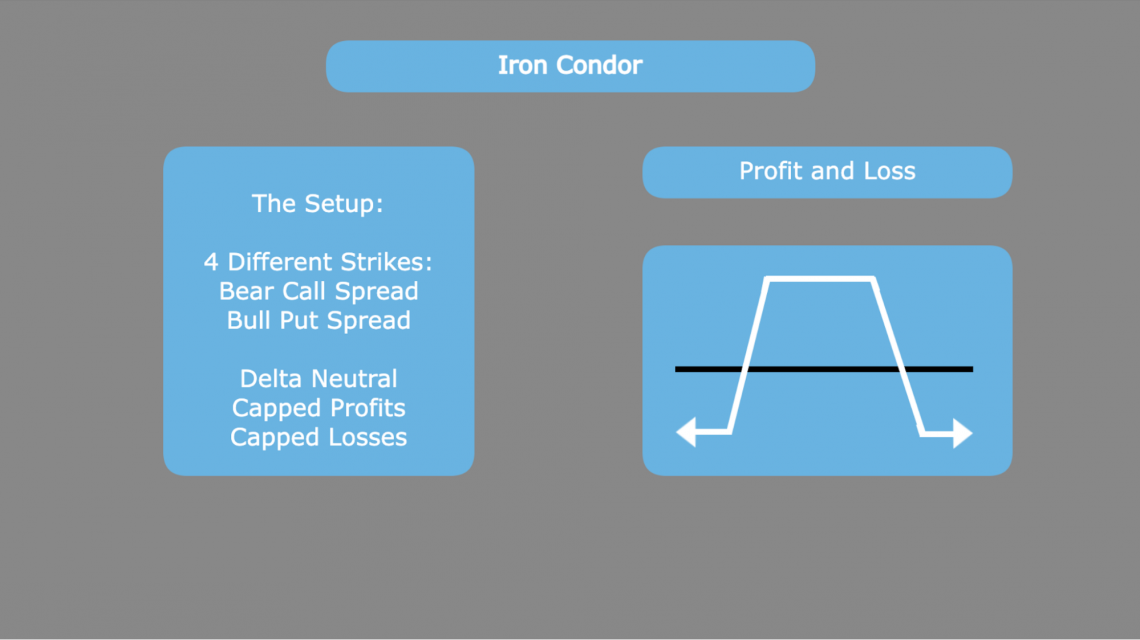The most common setup for this includes contracts that are all out-of-the-money. This means that a vertical put spread will be sold below the current market price and a vertical call spread will be sold above the current market price.

These two spreads are individually known as a bull put spread and a bear call spread. For the bull put spread, the goal is that the underlying expires at a price above the short put. The opposite is true for the bear call spread, which sees its greatest return when spot price is below the short call's strike.

Combining these two spreads, it is clear that the iron condor's profit is maximized when the market price of the underlying stock falls between the two middle strikes at expiration. This will lead to a situation where both spreads reach their individual maximum profits.

Because investors want the price to fall within this range, it is said that they are expecting low volatility. A stock moving significantly would mean that the stock is more likely to expire outside of the two strikes, and, perhaps, far enough to leave the investor with a loss.

Although options traders looking to implement the iron condor as an investment strategy are typically neutral, it is also possible to construct the strategy in a slightly bullish or bearish way.

By creating it in a way that the middle two strikes are either below or above the current price, the investor would move their maximum profit range either up or down. The conviction would, therefore, be slightly bullish or bearish, respectively.

In short, the iron condor is a strategy that:

• Is delta neutral

• Bets on low volatility

• Has capped potential for both profits and losses

As was mentioned before, the strategy as a whole is dependent on being delta neutral. This means that the investor does not pick which direction the stock moves to be profitable. A combination of matching vertical spreads achieves this goal.

For the iron condor, there are two vertical spreads used. Individually, both of these strategies are examples of credit spreads. This means that the two legs result in a net premium collected because the short legs are more expensive than the long legs. These spreads include: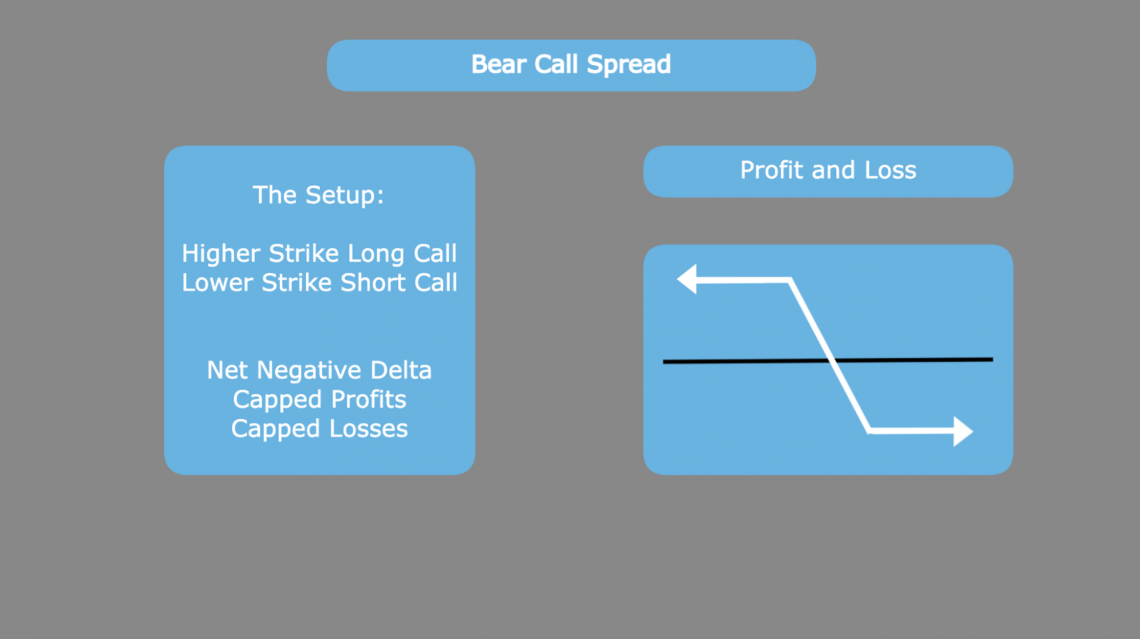The bear call spread strategy on its own is a moderately bearish bet. In this strategy, the investor opens a long call at a lower strike and opens a short call at a higher strike.

The combination of these two option legs creates a net negative delta, as the higher-strike short call will always be farther from the money, or less in the money, than the lower-strike long call.

The strategy itself has both a maximum profit and loss. The profit is limited to the premium initially collected. This total premium is also reduced by the long call, which is opened to hedge any risk that the short position creates.

The maximum profit of a bear call spread strategy is the total premium collected from selling the spread as a whole. The maximum loss is the strike difference multiplied by 100, minus the total premium collected to enter the position.

The investor's goal is, therefore, for the price to remain below the short leg, where the profit remains maximized.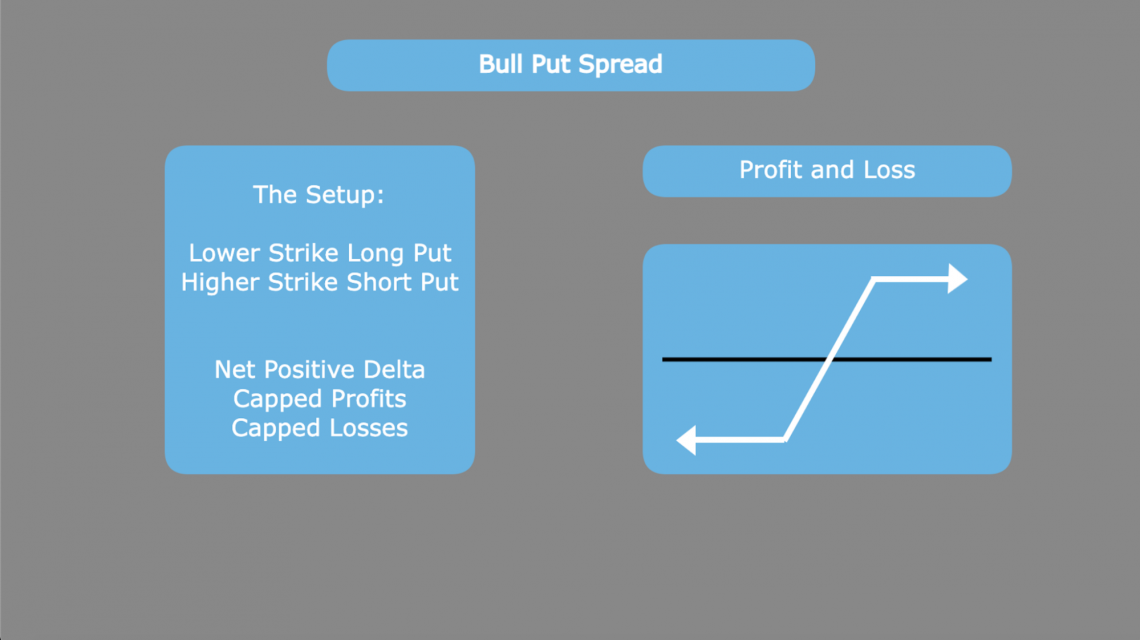The bull-put spread strategy on its own is a moderately bullish bet. In this strategy, the investor opens a long put at a lower strike and opens a short put at a higher strike.

The combination of these two option legs creates a net positive delta, as the lower-strike long put will always be farther from the money, or less in the money, than the higher-strike short put.

The strategy itself has both a maximum profit and loss. The profit is limited to the premium initially collected. This total premium is also reduced by the long put, which is opened to hedge the risk that the short position creates.

The maximum profit of a bull put spread strategy is the total premium collected from selling the spread as a whole. The maximum loss is the strike difference multiplied by 100, minus the total premium collected to enter the position.

The investor's goal is, therefore, for the price to remain above the short leg, where the profit remains maximized.

## Iron Condor Example

For this example, stock XYZ is currently trading at a price of 100. The following call and put options are expiring in 30 days with an implied volatility of 30.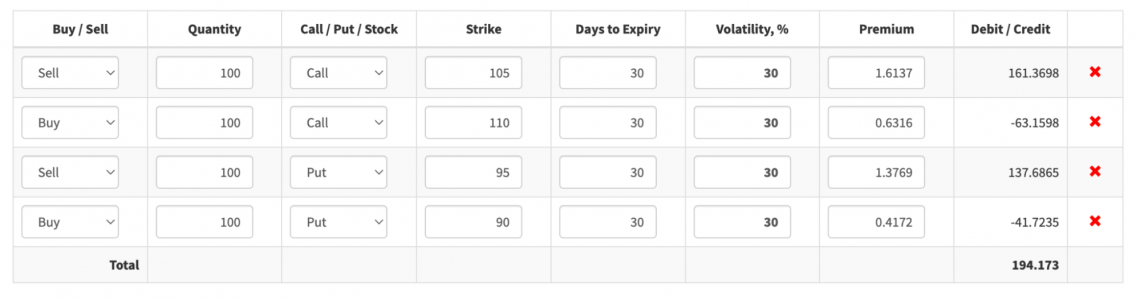Looking at the profit and loss graph, we can see that the maximum profit lies between the middle strikes of 95 and 105.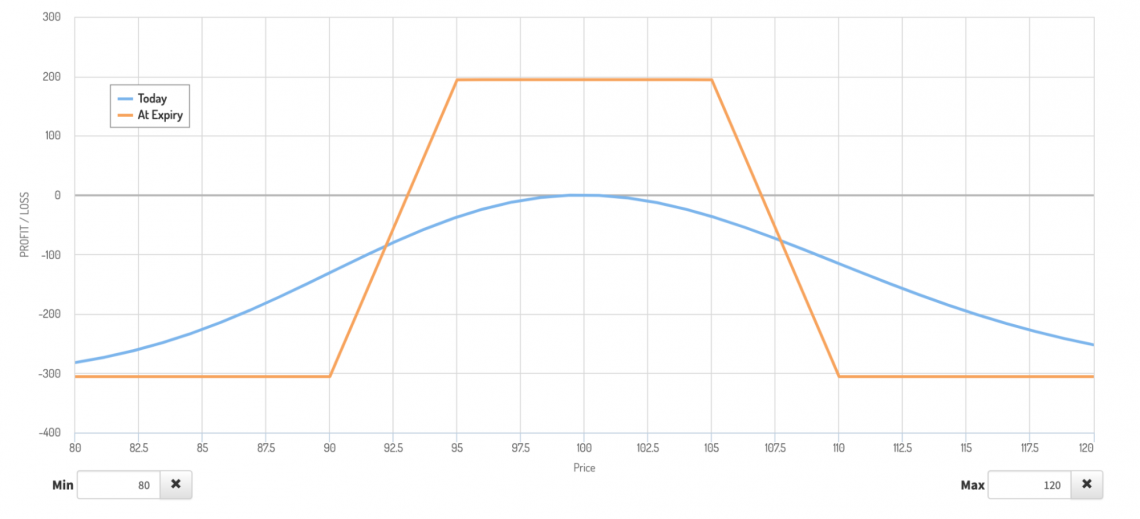At any point between these two strikes, the profit is simply the net premium collected from the four different contract legs. The premium collected will always be equal to the sum of individual premiums for the respective call and put spreads that are included in the contract (with net premium including any losses from either spread).

In this example, the net premium is \$194.17. If we look at the bear call spread and the bear put spread components, we see that the premiums are \$98.21 and \$95.96, respectively.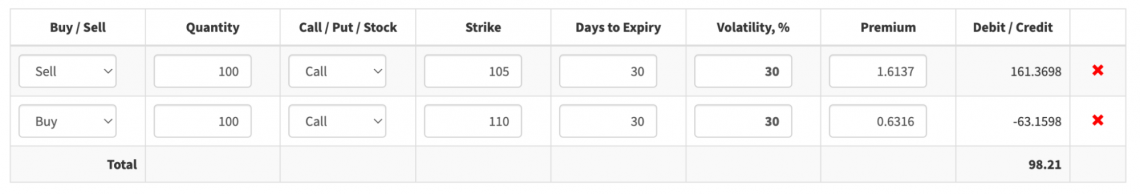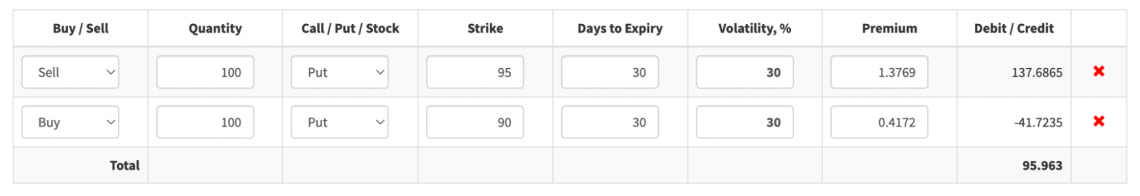When added together, these two premiums give us the total premium for the iron condor. Our example checks out.

As is the case with vertical credit spreads, the maximum loss and the break-even points are determined by the premium.

In this case, the maximum loss would occur at either point where the long call or long put becomes in the money. This is the point at which the long options begin to cover the short contract obligation, and, hence, stop the losses.

Looking at a situation where the underlying drops to \$88, we see the iron condor reaches its maximum loss because the embedded bull put spread reaches its maximum loss.Looking at the bull put spread, we see a maximum loss of \$404.04. This is equal to the difference between the strikes, multiplied by 100 (as each contract controls 100 shares), minus the premium collected. In this case, \$500 - \$95.96 gives us our max loss.

Our iron condor, however, has a loss of less than \$404.04. This is because the bear call spread expired out of the money. The additional premium from that option brings us to our actual loss of \$305.83.Looking closely, we can see that this is actually equal to the difference between the strikes and the premium collected as well. In this case, however, the extra premium collected from the bear call spread helps to lower the loss overall.

Max Loss = Strike Difference x 100 - Contract Premium

What is the downside of collecting this extra premium? At specific points, if the price rises too high, the bear call spread is in the money. This means that there is also a potential for losses if the price moves up instead of just if it moves down.

Looking past the long call \$110 strike, we see where the bear call spread reaches its maximum loss.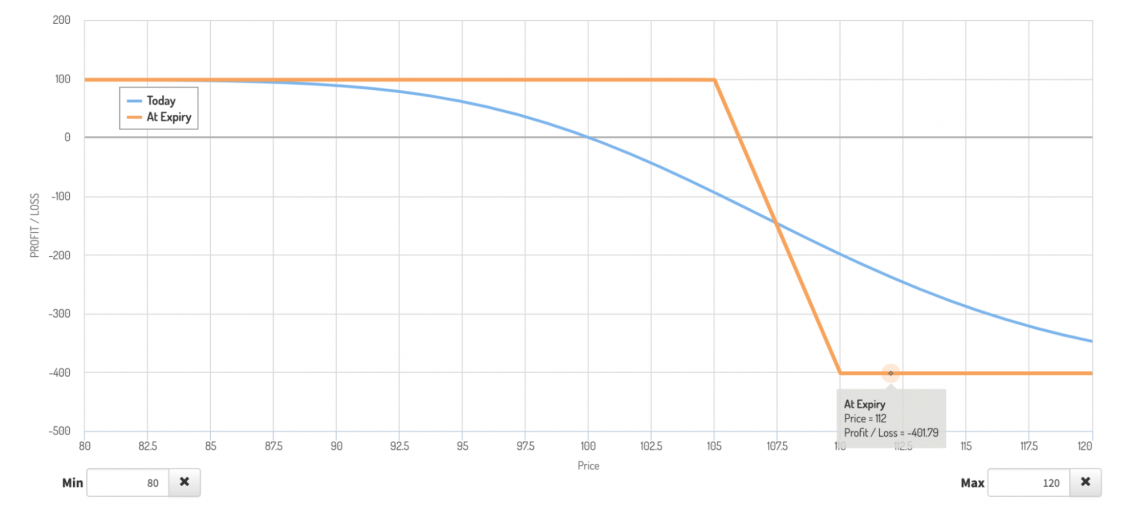The strike difference here is, once again, leaving the investor with a \$500 loss, which is partially offset by the \$98.31 in premium collected.

As was the case in the situation with a big price decline, the loss on the in-the-money spread is further offset by the premium of the opposing spread. In this case, the bull put spread is out of the money and helps chip away the loss of the bear call component.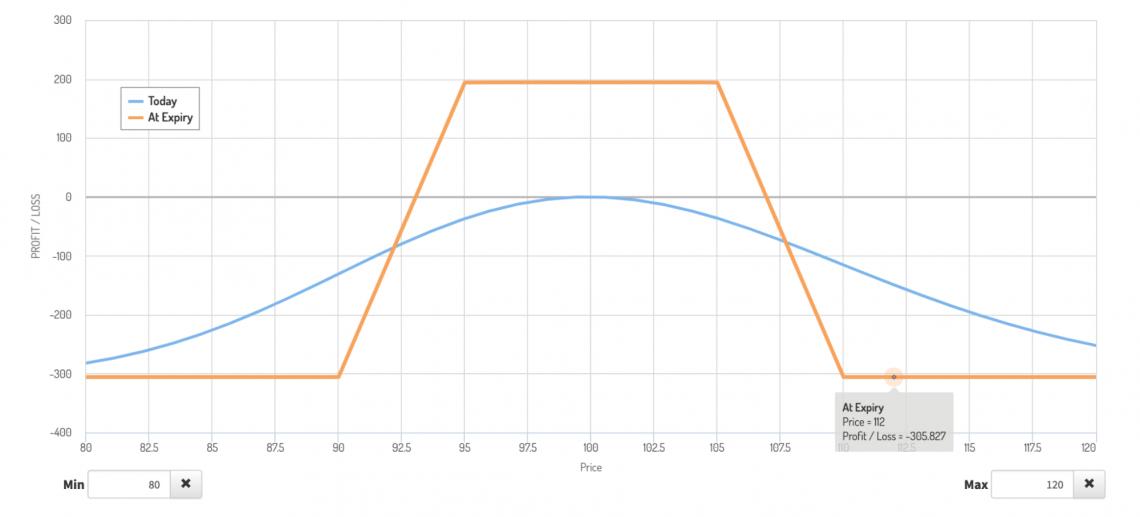Regardless of the direction of price movement, there is no situation where both vertical spreads are in the money. For this reason, the maximum loss is smaller, but there is a chance for a loss regardless of the price movement's direction.

## Final Considerations

While the key points regarding iron condors can be well summarized in a few points, investors looking to use this strategy must be aware of some other potential risks and factors that can affect this strategy's profitability.

First, the key points:

• It is a delta-neutral strategy, investors do not need a directional conviction on the underlying asset.

• Both the profit potential and loss potential for the strategy are fixed.

• This strategy involves four legs that can be broken down into two distinct vertical spreads.

Beyond these basic points, there are a few other aspects that can affect the profitability of the iron condor strategy. These aspects must be taken into account before entering a position.

The most important of these is the difference between actual volatility and implied volatility. Every option contract has implied volatility, which can be determined by the contract price.

Supply and demand for option contracts typically make implied volatility reflect the likelihood of actual movement relatively well.

Essentially, there is more demand for options with moving underlying because there is a greater chance of profit, increasing demand, and increasing price (due to the lack of an equal change in supply.

In situations where the investor is betting on low volatility, such as the iron condor, investors would be advised to consider how the implied volatility of the contract compares to their actual volatility expectations.

Higher volatility contracts will fetch a greater premium but are also, therefore, more likely to move outside of the middle strike (profit) range.

Investors would be smart to enter an iron condor (or any other delta-neutral position) when they think that the implied volatility of the contract is higher than the volatility they themselves expect during the contract's lifespan.

Selling a contract with high actual volatility that is likely to have its implied volatility lowered in the future will generate an extra premium while offering less risk in the future.

Beyond understanding volatility and its implications on price, traders must be aware of the early assignment risk present with short option legs. This is especially important when there is a dividend before expiration.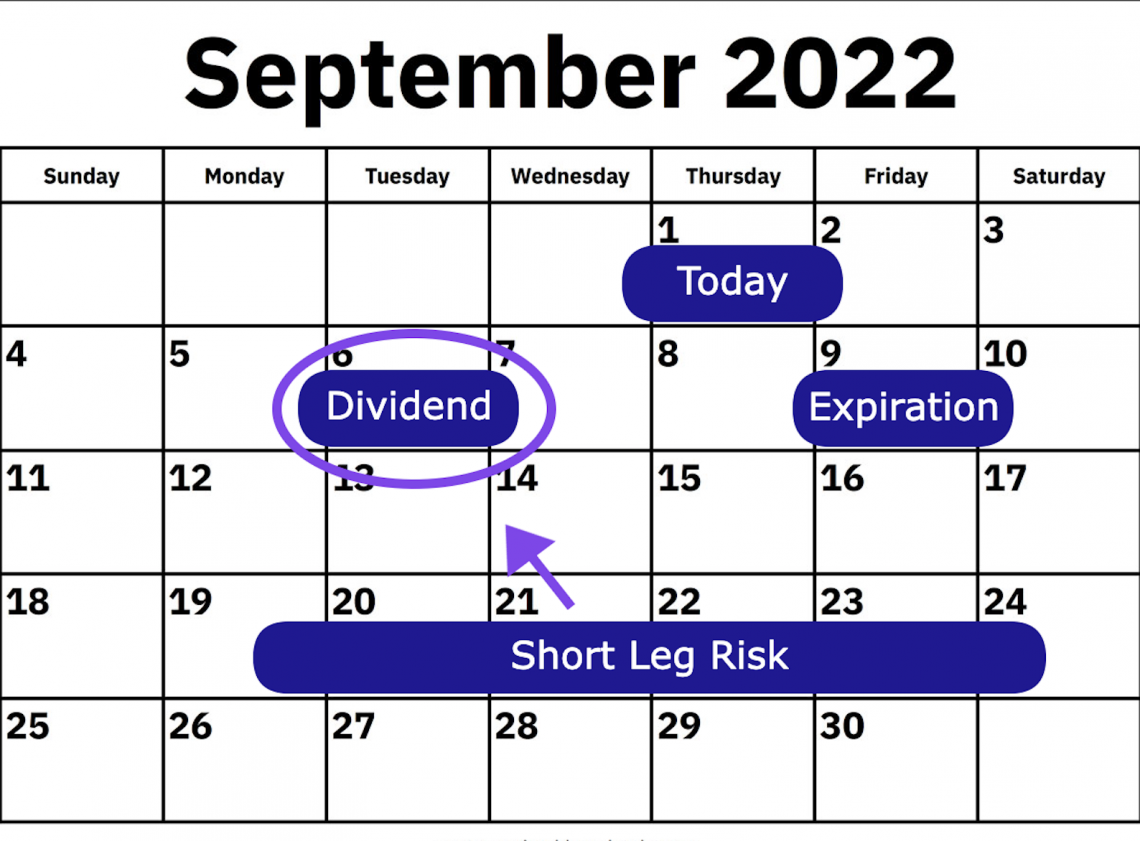This is because call option holders may decide to exercise if they think the dividend payment will be greater than the remaining time value in the contract. Conversely, put option holders may decide to exercise if they think the time value is greater than the dividend.

Depending on the account specifications, an early assignment can be quite impactful. If there is an insufficient balance in the account, liquidation may occur. This is a very real and extreme risk and has caused some traders to lose thousands of percent on their positions.

As with all options strategies, there is a greater degree of risk associated with increased leverage when using an iron condor. Beyond just understanding the setup, investors should have an understanding of equity valuation based on fundamentals.

For this strategy, it is fair to say that an investor thinks a stock will not have a drastic move in price. An investor may come to this conclusion if they feel that the fair value of the firm, based on its fundamentals, is equal to the current market price of the stock.Feel free to check out our financial modeling course to better understand these concepts. Due diligence on the underlying and a strong conviction of price movement is a must-have when using strategies susceptible to extra volatility.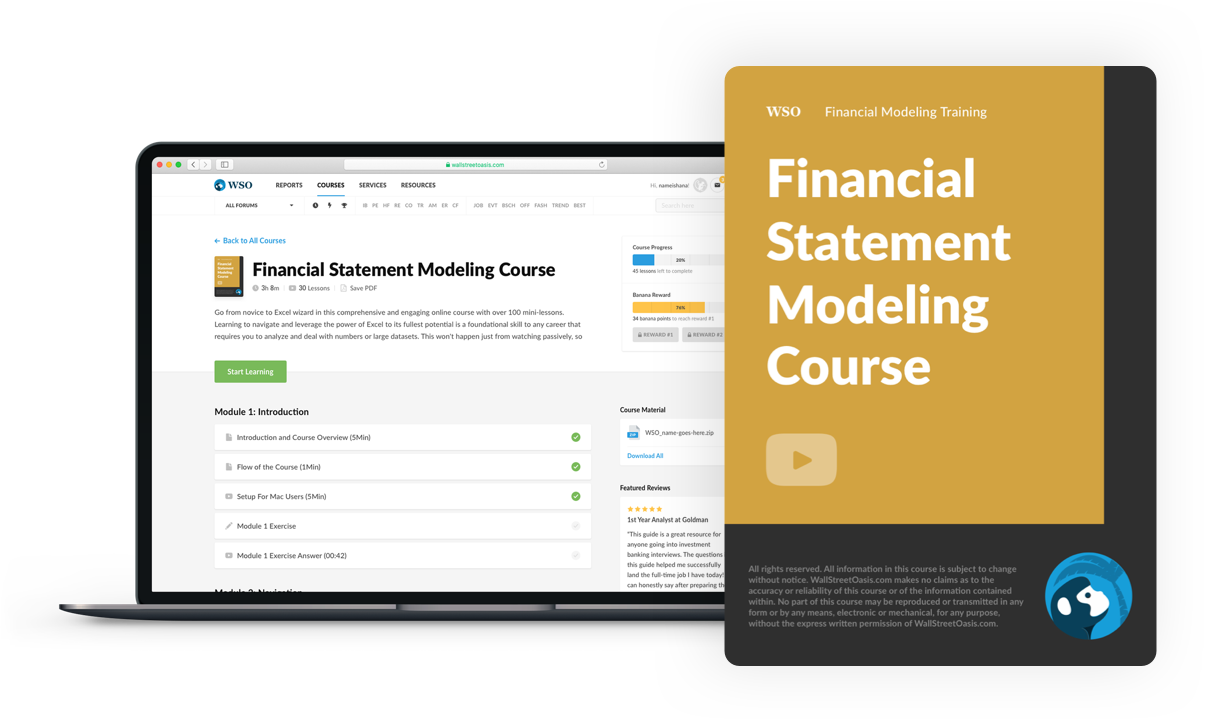### Everything You Need To Master Financial Statement Modeling

To Help You Thrive in the Most Prestigious Jobs on Wall Street.

Researched and Authored by Jacob RoundsLinkedIn

Reviewed and edited by James Fazeli-SinakiLinkedIn